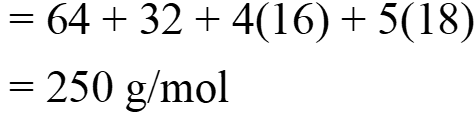Calculate the percentage of water of crystallization in CuS O 4 .5 H 2 O ( H ( =1, O =1, =16, S =16, = 32, Cu = 32, = 64).

# Calculate the percentage of water of crystallization in ( ( =1, =1, =16, =16, = 32, = 32, = 64).

1. A
40%
2. B
50%
3. C
36%
4. D
46%

Fill Out the Form for Expert Academic Guidance!l

+91

Live ClassesBooksTest SeriesSelf Learning

Verify OTP Code (required)

### Solution:

Concept: Copper sulphate is hygroscopic by nature. When copper sulphate and water interact, a hydrated version of the salt is created. Pentahydrate copper sulphate is created when copper sulphate combines with five water molecules.
The molecular weight of waterThe Molecular weight ofthe Percentage of water of crystallization-Hence, the percentage of water of crystallization is 36%.
Hence, the correct answer is option 3.

## Related content

 What Are The Career Options After 12th with PCM? Is Rajju Bhaiya University Recognized by The University Grants Commission? Golden Opportunity with Sri Chaitanya’s Score Scholarship Exam 2023 100 Paryayvachi Shabd (पर्यायवाची शब्द) – Paryayvachi Shabd in Hindi (Synonyms) Watts to horsepower conversion Infinity Learn: Rankguru Technology Solutions Private Limited BOD Full Form: Biochemical Oxygen Demand GMT Full Form: Greenwich Mean Time Manure and fertilisers Kidney+91

Live ClassesBooksTest SeriesSelf Learning

Verify OTP Code (required)